# Lions Weekly: February 15, 2023# A Note From Mr. Sandefur

Lion Family,

It has been a very special few weeks on campus as we have experienced a wonderful re-enrollment season, incredible success in athletics (basketball and cheerleading), impactful service projects, and meaningful relationships being developed. On top of all of this excitement, our students celebrated Valentine's this week as well. As the world focuses on sharing love one day a year, it is our desire to share the love of Christ daily with all we come in contact with. It is only because of the love of God that we are able to do what we do.

One of the most unique aspects of The King's Academy is our faith-based international boarding program which has been a hallmark of The Academy for many years. Because of the faithful support of so many, there are students from many nations (China, Brazil, Aruba, Curacao, Sudan, Rwanda, Czech Republic, Bonaire, New Guinea, Japan, Montenegro, Italy, Hong Kong, and soon to be Kenya) that are able to receive the love of Christ through word, action, and service. Please join me in praying that students come to know the saving love of Christ as we seek to faithfully serve our community and the world.

Jeremy Sandefur

 table div table+table+table+table div table{width:100%;padding:0}table div table+table+table+table div table img{width:96.23%;padding:0;float:none}table div table+table+table+table div table td{width:100%;padding:0 1.88% 18px}/* styles */table div table+table+table+table+table+table div table{width:100%;padding:0}table div table+table+table+table+table+table div table img{width:96.23%;padding:0;float:none}table div table+table+table+table+table+table div table td{width:100%;padding:0 1.88% 18px}/* styles */# Upcoming Mandatory Meetings

Save the date and mark your calendar for these upcoming mandatory meetings for the Washington D. C. trip and Europe Trip.table div table+table+table+table+table+table+table+table+table div table{width:100%;padding:0}table div table+table+table+table+table+table+table+table+table div table img{width:96.23%;padding:0;float:none}table div table+table+table+table+table+table+table+table+table div table td{width:100%;padding:0 1.88% 18px}/* styles */table div table+table+table+table+table+table+table+table+table+table+table div table{width:100%;padding:0}table div table+table+table+table+table+table+table+table+table+table+table div table img{width:96.23%;padding:0;float:none}table div table+table+table+table+table+table+table+table+table+table+table div table td{width:100%;padding:0 1.88% 18px}/* styles */table div table+table+table+table+table+table+table+table+table+table+table+table+table div table{width:100%;padding:0}table div table+table+table+table+table+table+table+table+table+table+table+table+table div table img{width:96.23%;padding:0;float:none}table div table+table+table+table+table+table+table+table+table+table+table+table+table div table td{width:100%;padding:0 1.88% 18px}/* styles */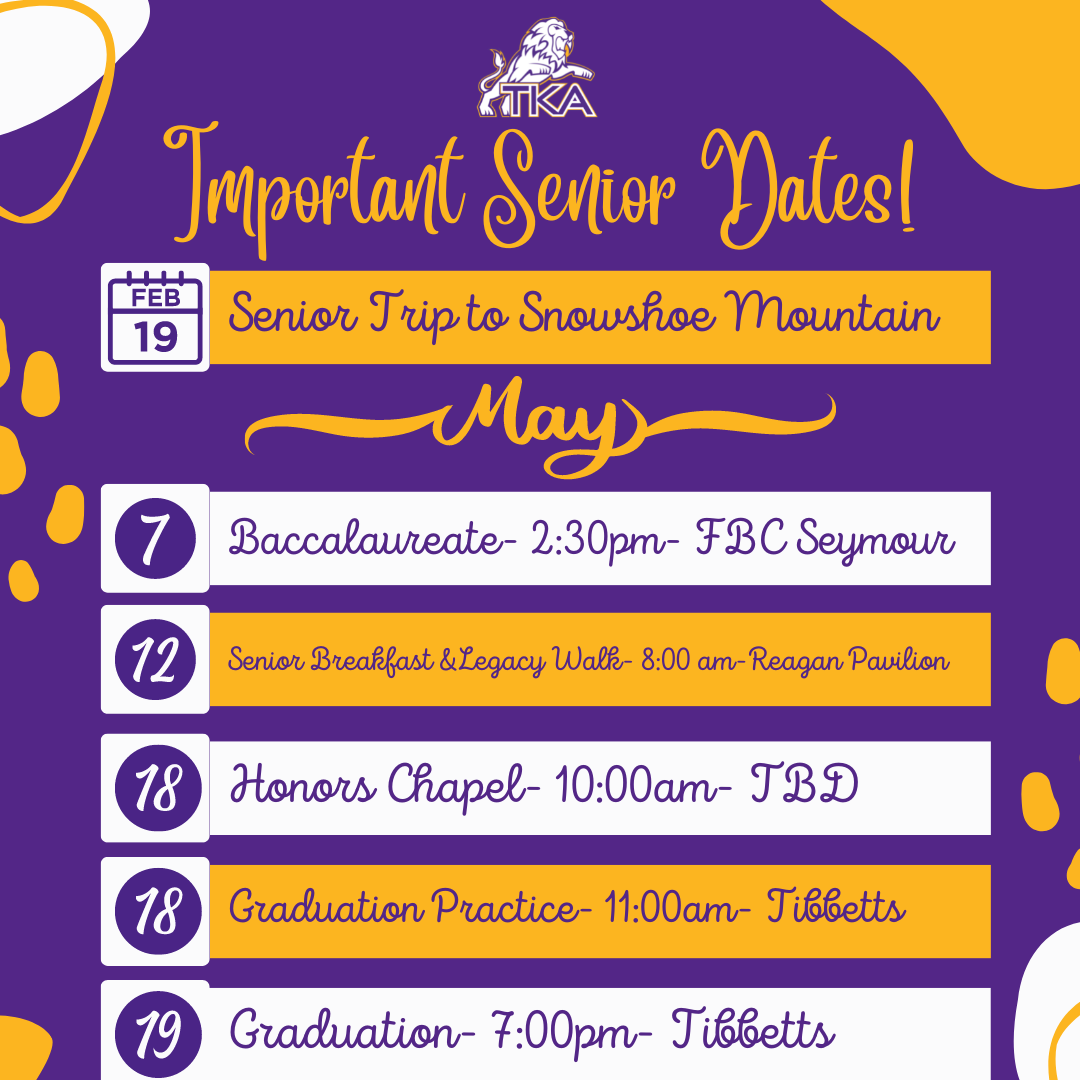table div table+table+table+table+table+table+table+table+table+table+table+table+table+table+table div table{width:100%;padding:0}table div table+table+table+table+table+table+table+table+table+table+table+table+table+table+table div table img{width:96.23%;padding:0;float:none}table div table+table+table+table+table+table+table+table+table+table+table+table+table+table+table div table td{width:100%;padding:0 1.88% 18px}/* styles */# Host Family Informational Meeting

## Thursday, February 16

TKA is excited about this new opportunity to partner with Faces & Our Cultures to bring students from Guatemala for 7 weeks to The Academy.

Middle School students will stay with host families for the entire 7 weeks. High School students will stay in the dorm during the week and with host families on the weekend.

The young cultural ambassadors will arrive on Saturday, October 14th, stay with their host families and join our school community for the following seven (7) weeks, and then depart on Saturday, December 9th.

If you are potentially interested in hosting a student and participating in this life-enriching experience, or would like more information, please plan on attending the informational meeting on Thursday, February 16, at 8:00 a.m. in the Clark Library.

If you have any additional questions, please feel free to contact Ms. Janice Mink (jmink@thekingsacademy.net) or Sandy Cardona (sandy.cardona@facesandcultures.com).

 table div table+table+table+table+table+table+table+table+table+table+table+table+table+table+table+table+table div table{width:100%;padding:0}table div table+table+table+table+table+table+table+table+table+table+table+table+table+table+table+table+table div table img{width:96.23%;padding:0;float:none}table div table+table+table+table+table+table+table+table+table+table+table+table+table+table+table+table+table div table td{width:100%;padding:0 1.88% 18px}/* styles */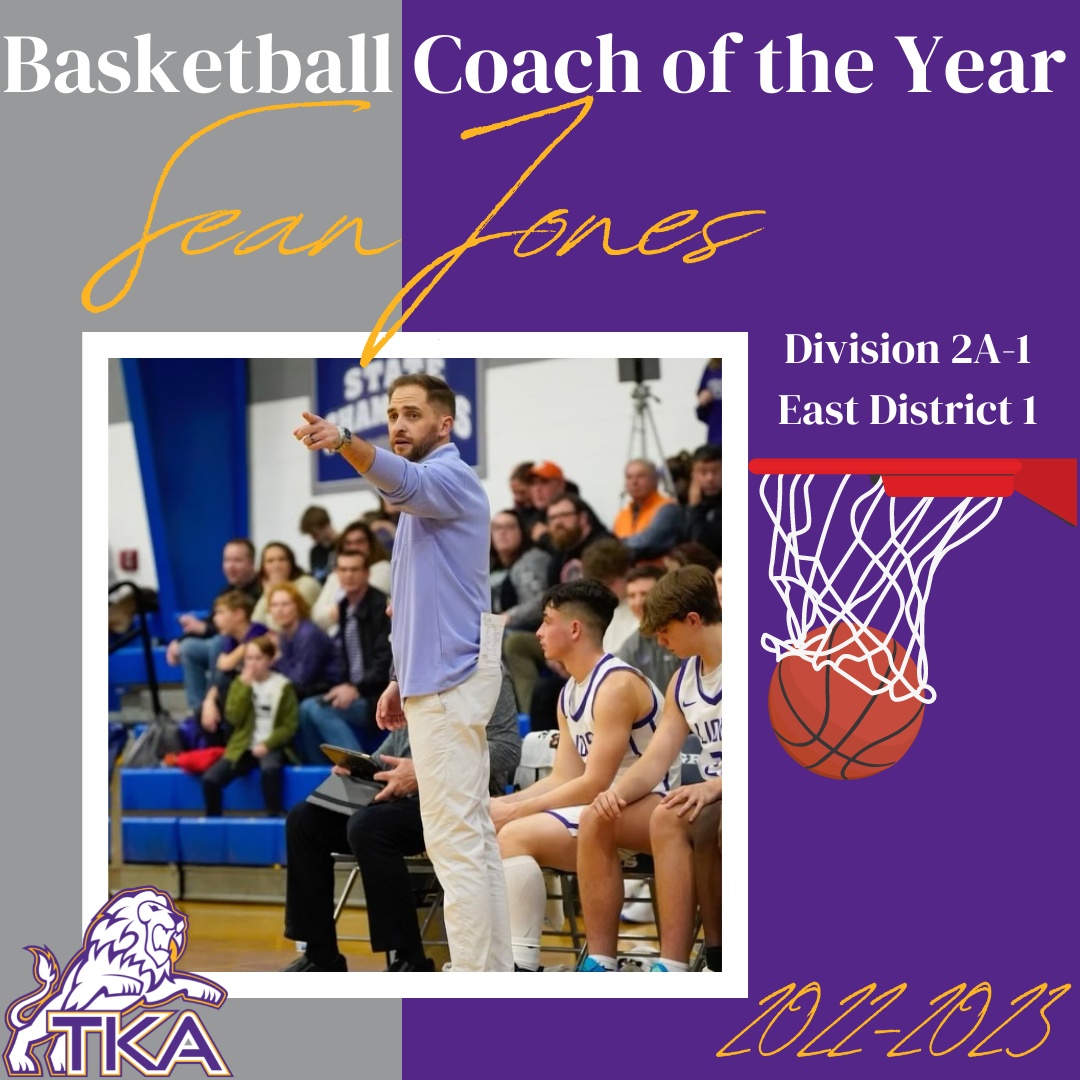# Basketball Coach of the Year

## Sean Jones

Congratulations to Coach Jones for being named Division 2A-1 East District 1 Coach of the Year! Coach Jones has led our varsity boys' basketball through the district championship and is now in the regional playoff games. We are looking forward to seeing all this team will accomplish this year under Coach Jones' leadership. Congratulations, Coach Jones!## District Runner-Up

Our Lady Lions have worked hard this season to be the Division 2A-1 East District 1 Runner-up! They are continuing to represent TKA well as they play in the regional games.

Make plans to join them on Friday night at 6:30 in Lakeway's gym for the semi-final regional playoffs.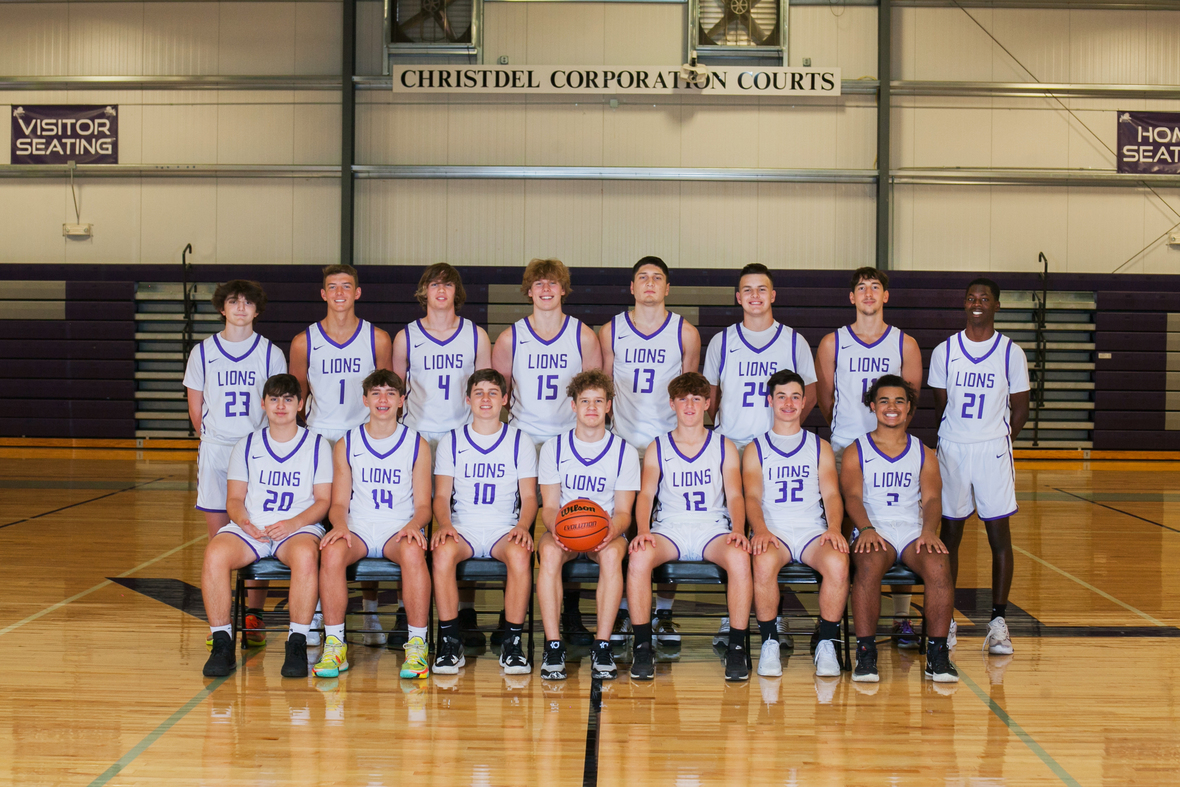## District Champions

The Varsity Boys have worked hard this season as well to be the Division 2A-1 East District 1 Champions! They are continuing to play hard and represent TKA well in the regional playoffs.

Make plans to join them on Friday night at 5:00 in Lakeway's gym for the semi-final regional playoffs.

 table div table+table+table+table+table+table+table+table+table+table+table+table+table+table+table+table+table+table+table+table+table+table div table{width:100%;padding:0}table div table+table+table+table+table+table+table+table+table+table+table+table+table+table+table+table+table+table+table+table+table+table div table img{width:96.23%;padding:0;float:none}table div table+table+table+table+table+table+table+table+table+table+table+table+table+table+table+table+table+table+table+table+table+table div table td{width:100%;padding:0 1.88% 18px}/* styles */# Elementary Super Reader Week 2023

We are excited to share with you about our 2nd Annual Super Reader Week! Super Reader Week will take place February 27-March 3rd. This week coincides with Read Across America Week highlighting Dr. Seuss’ birthday. We have planned a fun-filled week highlighting the great importance of reading.

Super Reader Week will include a Dr. Seuss environment throughout the elementary with decorated doors and hallways, Dr. Seuss Dress Up Days, a special reading time with a Super Reader during library class, Special Super Readers throughout the week in class, Dr. Seuss activities, and a Reading Celebration Day. Also, students will have opportunities to purchase books, book tools, and other fun items in our Book Fair located in our school library.

As we work together to create a bigger emphasis on reading this year, we want you to get involved! We have set an elementary goal for our Lions to read 25,000 minutes during super reader week. This reading goal will begin on Wednesday, February 22nd and continue until Thursday, March 2nd. To accomplish this goal, each Lion will need to read a minimum of 20 minutes every night with a family member. As your Lion reads with you each night, be sure to sign the super reader week reading log so we can track our elementary-wide progress throughout the week. Once our Lions meet their reading goal, we will celebrate with a special surprise on Friday.

It is a joy to be a part of a school that takes great pride in developing strong readers. We are beyond blessed to share our love for reading with your Lion every day. We are looking forward to a super fun week celebrating our little lion readers.

 /* styles */ Blessings, Kelly Sullivan Elementary Principal

# Elementary Super Reader Week Dress Up Days# Monday, February 27

## Oh, the Places You’ll Go

Dress in your favorite college team/jersey with jeans or in your normal school uniform.

Remember, all attire must adhere to dress code.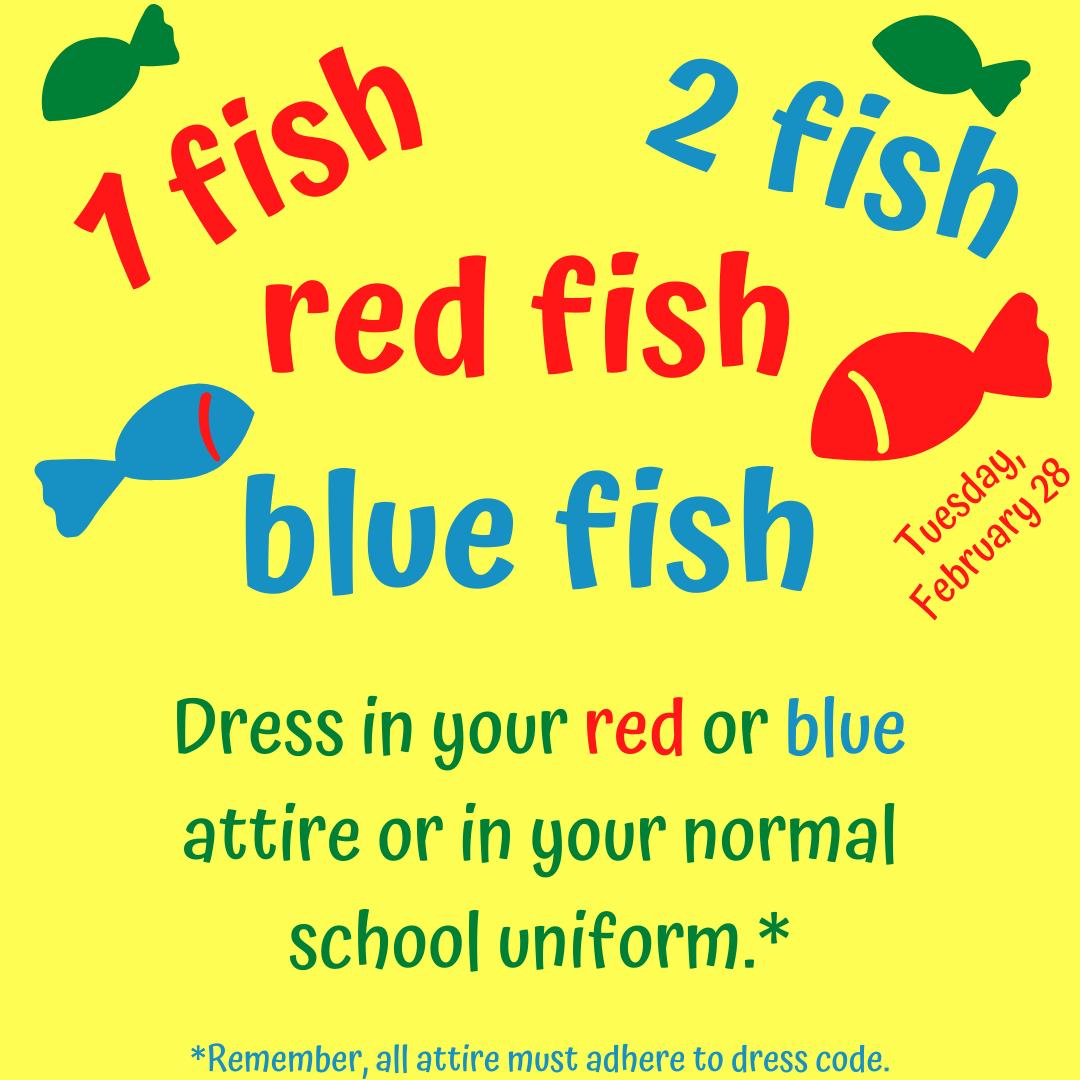# Tuesday, February 28

## One Fish, Two Fish, Red Fish, Blue Fish

Dress in your red or blue attire or in your normal school uniform.

Remember, all attire must adhere to dress code.# Wednesday, March 1

## Wacky Wednesday

Wear your clothes backward, inside out, or mismatched or your normal school uniform.

Remember, all attire must adhere to dress code.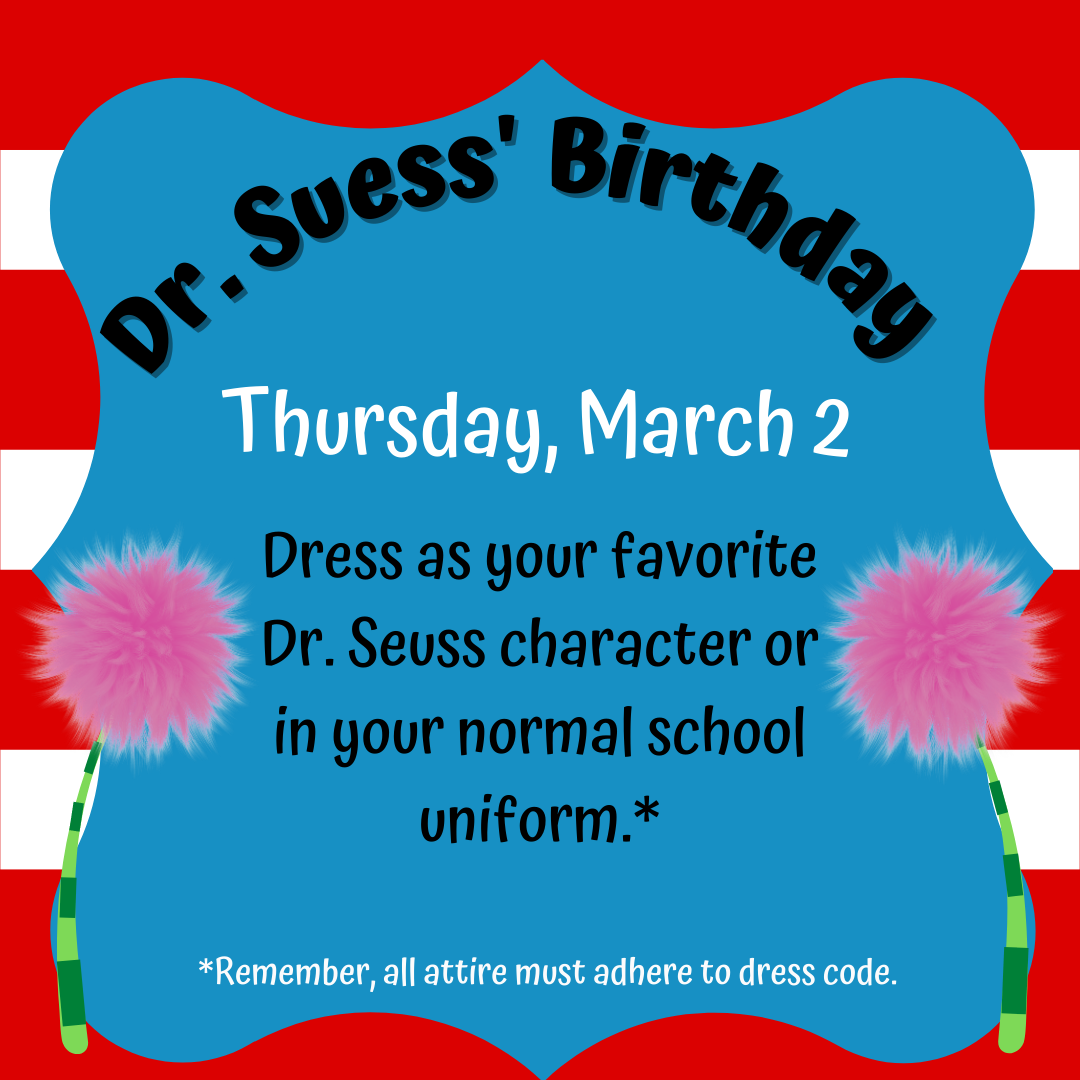# Thursday, March 2

## Dr. Suess' Birthday

Dress as your favorite Dr. Seuss character or in your normal school uniform.

Remember, all attire must adhere to dress code.# Friday, March 3

## The Sleep Book

Remember, all attire must adhere to dress code.table div table+table+table+table+table+table+table+table+table+table+table+table+table+table+table+table+table+table+table+table+table+table+table+table+table+table+table+table+table+table+table+table+table+table+table div table{width:100%;padding:0}table div table+table+table+table+table+table+table+table+table+table+table+table+table+table+table+table+table+table+table+table+table+table+table+table+table+table+table+table+table+table+table+table+table+table+table div table img{width:96.23%;padding:0;float:none}table div table+table+table+table+table+table+table+table+table+table+table+table+table+table+table+table+table+table+table+table+table+table+table+table+table+table+table+table+table+table+table+table+table+table+table div table td{width:100%;padding:0 1.88% 18px}/* styles */# Familiar Faces In New Places: Academic Changes for 2023-2024 School Year# Kendedrick Jones - High School Principal and Director Residence Life

Mr. Kendedrick Jones will be transitioning to the role of High School Principal in June of 2023. Mr. Jones' experience as a former High School Science teacher, principal, and administrator will be beneficial as he leads our High School faculty in continued growth, new offerings, and the launch of our new bio-medical sciences program (information coming soon). Mr. Jones will continue to oversee the Residence Life program, however in a slightly different manner as both Dormitory Directors will report to him for guidance.

Please join me in congratulating Mr. Jones on this exciting new opportunity as he serves our TKA family in new ways!# Blake Fuller - Middle School Principal and Director of Communications

Mr. Blake Fuller will be transitioning to a new role overseeing our Middle School as Principal. Mr. Fuller has faithfully served TKA as the MS/HS Principal and is looking forward to multiplying his impact by focusing on curating a deeply meaningful middle school experience for our students. Mr. Fuller is passionate about seeing our middle school students receive special attention and opportunities as they discover who God has created them to be.

Mr. Fuller's background as an English teacher provides a unique skill set as he will also serve as our Director of Communications.

We are grateful for Mr. Fuller's impact thus far on TKA and are looking forward to his service in these new areas.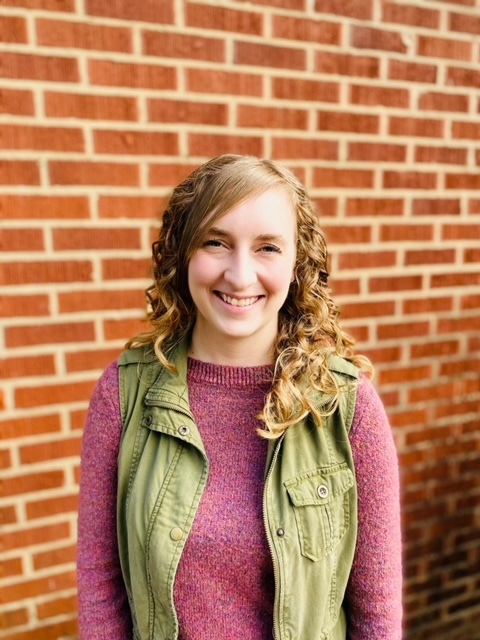# Kelly Clifton - Academic Dean

Mrs. Kelly Clifton will be transitioning from her role as Director of Communications and Academic Support to her new role as Academic Dean. Mrs. Clifton's new role will be a blend of academic support and advancement as she supports our principals and teachers in the daily application of all student support services, curriculum upgrades, biblical integration, and more.

Please join me in congratulating Mrs. Clifton on this exciting change as she helps our students and teachers reach new heights!

 table div table+table+table+table+table+table+table+table+table+table+table+table+table+table+table+table+table+table+table+table+table+table+table+table+table+table+table+table+table+table+table+table+table+table+table+table+table+table+table+table+table div table{width:100%;padding:0}table div table+table+table+table+table+table+table+table+table+table+table+table+table+table+table+table+table+table+table+table+table+table+table+table+table+table+table+table+table+table+table+table+table+table+table+table+table+table+table+table+table div table img{width:96.23%;padding:0;float:none}table div table+table+table+table+table+table+table+table+table+table+table+table+table+table+table+table+table+table+table+table+table+table+table+table+table+table+table+table+table+table+table+table+table+table+table+table+table+table+table+table+table div table td{width:100%;padding:0 1.88% 18px}/* styles */table div table+table+table+table+table+table+table+table+table+table+table+table+table+table+table+table+table+table+table+table+table+table+table+table+table+table+table+table+table+table+table+table+table+table+table+table+table+table+table+table+table+table+table div table{width:100%;padding:0}table div table+table+table+table+table+table+table+table+table+table+table+table+table+table+table+table+table+table+table+table+table+table+table+table+table+table+table+table+table+table+table+table+table+table+table+table+table+table+table+table+table+table+table div table img{width:96.23%;padding:0;float:none}table div table+table+table+table+table+table+table+table+table+table+table+table+table+table+table+table+table+table+table+table+table+table+table+table+table+table+table+table+table+table+table+table+table+table+table+table+table+table+table+table+table+table+table div table td{width:100%;padding:0 1.88% 18px}/* styles */# D1 Training

Middle/High school athletes can come train outside of their team training hours at the listed times.

For any additional information, parents can contact Mr. Melvin Jones.

Melvin Jones
D1 Sevierville
865-719-9421
melvin.jones@d1training.com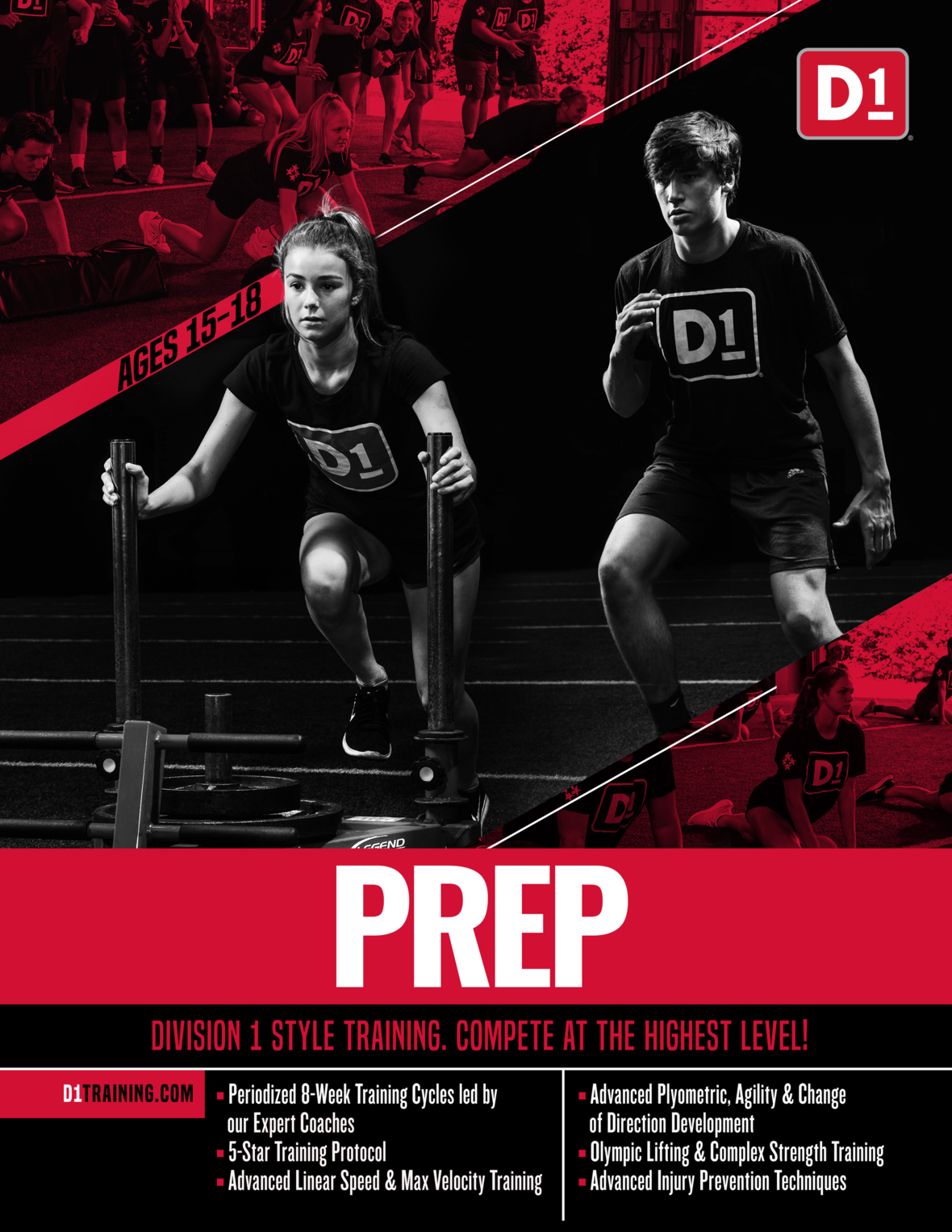table div table+table+table+table+table+table+table+table+table+table+table+table+table+table+table+table+table+table+table+table+table+table+table+table+table+table+table+table+table+table+table+table+table+table+table+table+table+table+table+table+table+table+table+table+table+table div table{width:100%;padding:0}table div table+table+table+table+table+table+table+table+table+table+table+table+table+table+table+table+table+table+table+table+table+table+table+table+table+table+table+table+table+table+table+table+table+table+table+table+table+table+table+table+table+table+table+table+table+table div table img{width:96.23%;padding:0;float:none}table div table+table+table+table+table+table+table+table+table+table+table+table+table+table+table+table+table+table+table+table+table+table+table+table+table+table+table+table+table+table+table+table+table+table+table+table+table+table+table+table+table+table+table+table+table+table div table td{width:100%;padding:0 1.88% 18px}/* styles */# 2022-23 Yearbooks

2022-23 yearbooks are now on sale! Scan this QR code to visit the site to purchase yours today. For any questions regarding yearbooks, contact Mr. Josh Smith at jsmith@thekingsacademy.net.

 table div table+table+table+table+table+table+table+table+table+table+table+table+table+table+table+table+table+table+table+table+table+table+table+table+table+table+table+table+table+table+table+table+table+table+table+table+table+table+table+table+table+table+table+table+table+table+table+table div table{width:100%;padding:0}table div table+table+table+table+table+table+table+table+table+table+table+table+table+table+table+table+table+table+table+table+table+table+table+table+table+table+table+table+table+table+table+table+table+table+table+table+table+table+table+table+table+table+table+table+table+table+table+table div table img{width:96.23%;padding:0;float:none}table div table+table+table+table+table+table+table+table+table+table+table+table+table+table+table+table+table+table+table+table+table+table+table+table+table+table+table+table+table+table+table+table+table+table+table+table+table+table+table+table+table+table+table+table+table+table+table+table div table td{width:100%;padding:0 1.88% 18px}/* styles */table div table+table+table+table+table+table+table+table+table+table+table+table+table+table+table+table+table+table+table+table+table+table+table+table+table+table+table+table+table+table+table+table+table+table+table+table+table+table+table+table+table+table+table+table+table+table+table+table+table+table div table{width:100%;padding:0}table div table+table+table+table+table+table+table+table+table+table+table+table+table+table+table+table+table+table+table+table+table+table+table+table+table+table+table+table+table+table+table+table+table+table+table+table+table+table+table+table+table+table+table+table+table+table+table+table+table+table div table img{width:96.23%;padding:0;float:none}table div table+table+table+table+table+table+table+table+table+table+table+table+table+table+table+table+table+table+table+table+table+table+table+table+table+table+table+table+table+table+table+table+table+table+table+table+table+table+table+table+table+table+table+table+table+table+table+table+table+table div table td{width:100%;padding:0 1.88% 18px}/* styles */# Weekly Sports Schedule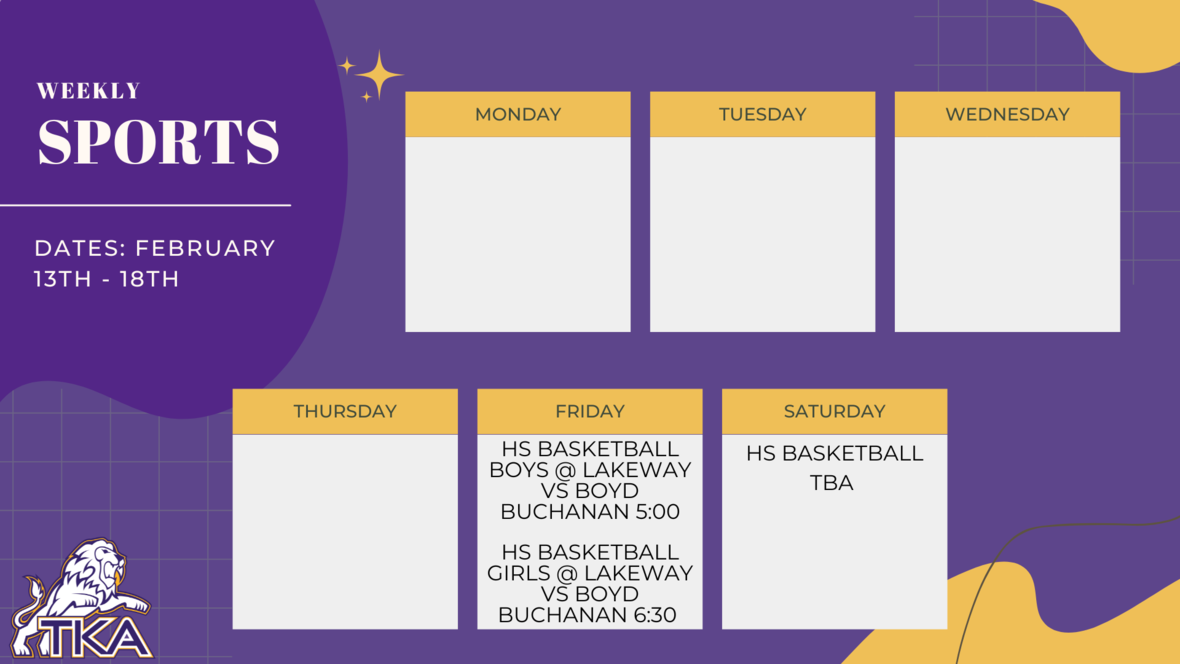table div table+table+table+table+table+table+table+table+table+table+table+table+table+table+table+table+table+table+table+table+table+table+table+table+table+table+table+table+table+table+table+table+table+table+table+table+table+table+table+table+table+table+table+table+table+table+table+table+table+table+table+table+table div table{width:100%;padding:0}table div table+table+table+table+table+table+table+table+table+table+table+table+table+table+table+table+table+table+table+table+table+table+table+table+table+table+table+table+table+table+table+table+table+table+table+table+table+table+table+table+table+table+table+table+table+table+table+table+table+table+table+table+table div table img{width:96.23%;padding:0;float:none}table div table+table+table+table+table+table+table+table+table+table+table+table+table+table+table+table+table+table+table+table+table+table+table+table+table+table+table+table+table+table+table+table+table+table+table+table+table+table+table+table+table+table+table+table+table+table+table+table+table+table+table+table+table div table td{width:100%;padding:0 1.88% 18px}/* styles */# Important information for the 2022-2023 School Year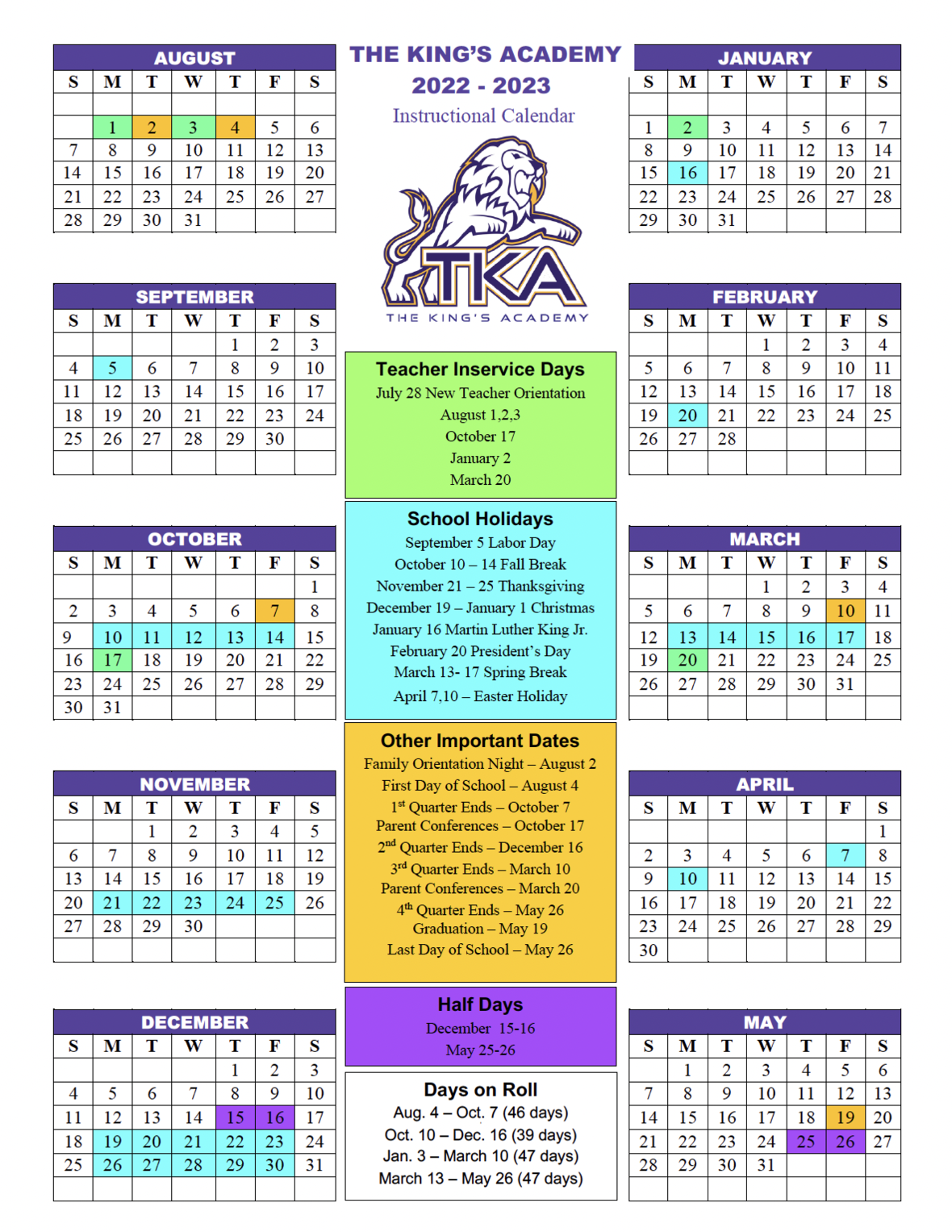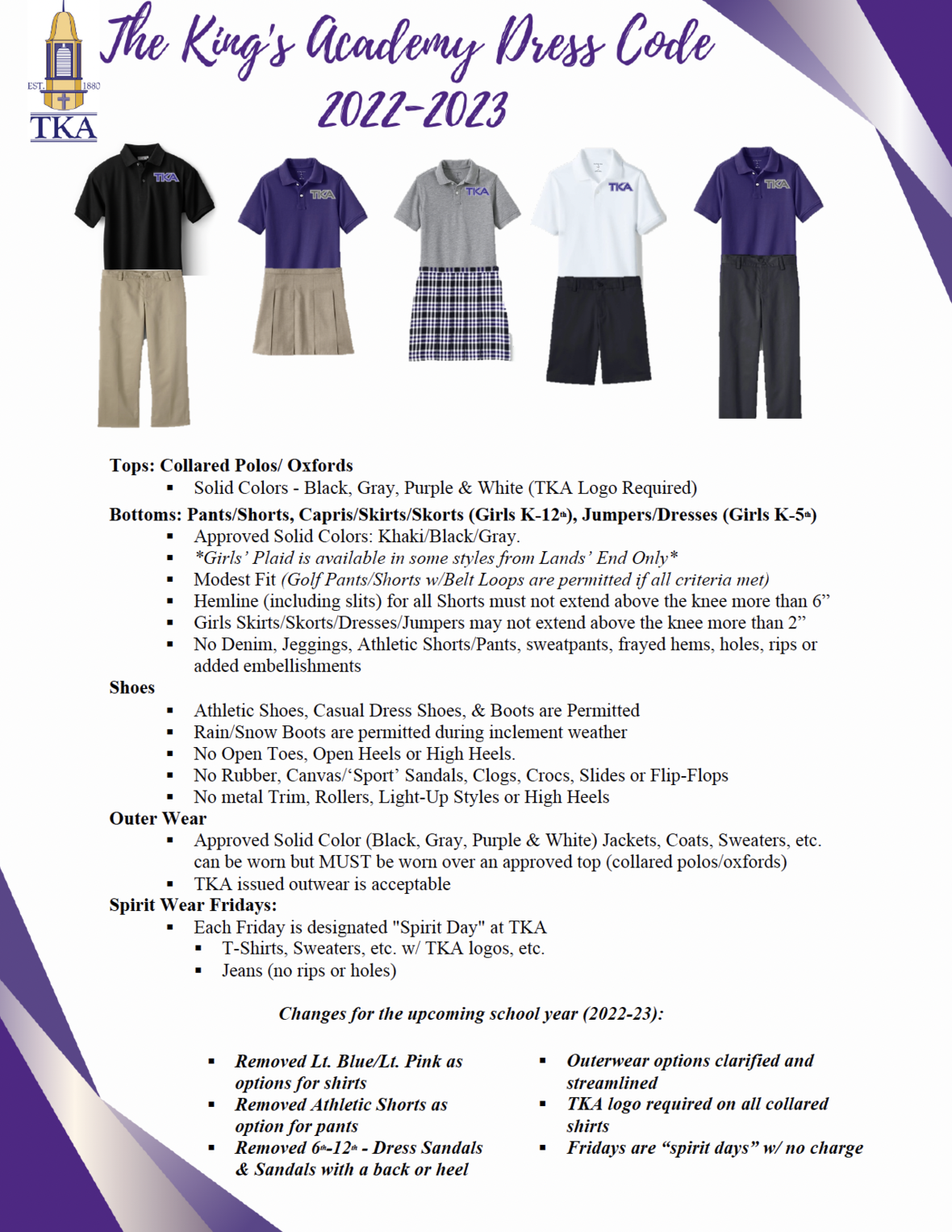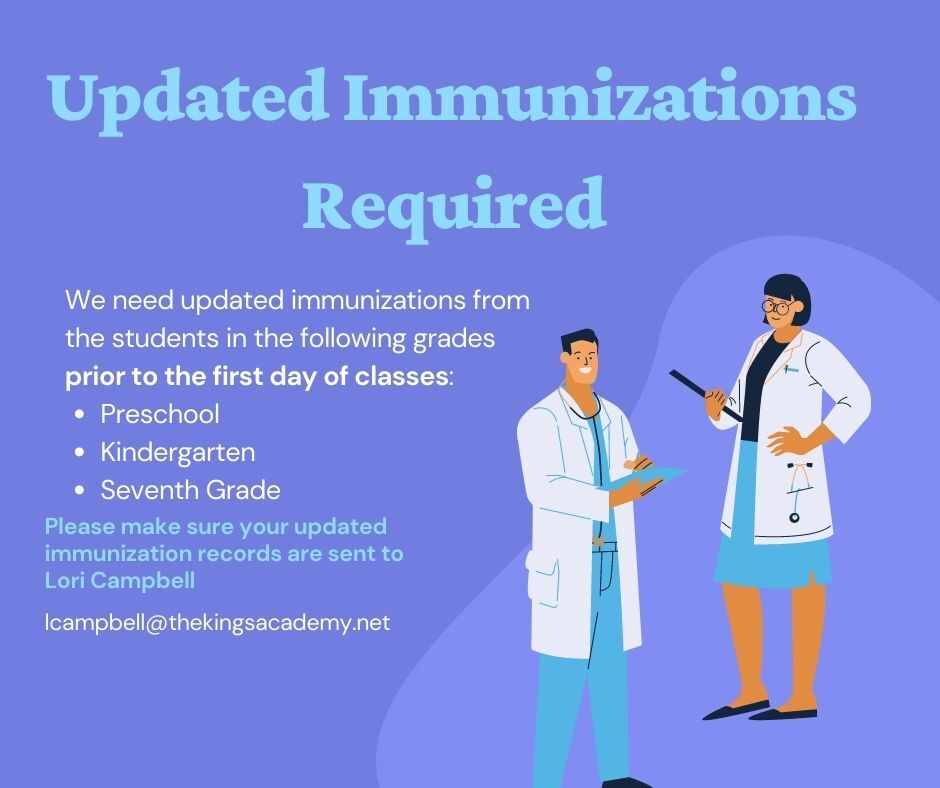table div table+table+table+table+table+table+table+table+table+table+table+table+table+table+table+table+table+table+table+table+table+table+table+table+table+table+table+table+table+table+table+table+table+table+table+table+table+table+table+table+table+table+table+table+table+table+table+table+table+table+table+table+table+table+table+table+table+table div table{width:100%;padding:0}table div table+table+table+table+table+table+table+table+table+table+table+table+table+table+table+table+table+table+table+table+table+table+table+table+table+table+table+table+table+table+table+table+table+table+table+table+table+table+table+table+table+table+table+table+table+table+table+table+table+table+table+table+table+table+table+table+table+table div table img{width:96.23%;padding:0;float:none}table div table+table+table+table+table+table+table+table+table+table+table+table+table+table+table+table+table+table+table+table+table+table+table+table+table+table+table+table+table+table+table+table+table+table+table+table+table+table+table+table+table+table+table+table+table+table+table+table+table+table+table+table+table+table+table+table+table+table div table td{width:100%;padding:0 1.88% 18px}/* styles */# TKA Visitor Policy

In order to help maintain a safe and instructionally based learning environment for our students, all visitors for middle and high school must enter through the front door of the Anderson Building and sign in in the middle/high school office in order to receive a visitor's pass. All visitors for elementary must enter the front door of the Ogle Building in order to receive a visitor's pass. This pass should be visible at all times so that TKA faculty and staff know that you have checked in at the office.

Our doors are open to visitors, but please call ahead of time to schedule conferences with your child’s teachers. This will prevent interruptions to instruction and the loss of the valuable learning time of our students. Adults will not be allowed to wander the halls of the building or hold conferences with teachers during instructional times.

TKA family lunch visitors should follow the above procedure. Lunch tickets are \$5 each.

Students who are not enrolled at TKA are not permitted to visit TKA students during the school day, which includes lunch.

This policy has been developed in order to help maintain a safe and instruction focused environment at school each day. Any visitor who does not meet these expectations may be asked to leave school grounds.

 table div table+table+table+table+table+table+table+table+table+table+table+table+table+table+table+table+table+table+table+table+table+table+table+table+table+table+table+table+table+table+table+table+table+table+table+table+table+table+table+table+table+table+table+table+table+table+table+table+table+table+table+table+table+table+table+table+table+table+table+table div table{width:100%;padding:0}table div table+table+table+table+table+table+table+table+table+table+table+table+table+table+table+table+table+table+table+table+table+table+table+table+table+table+table+table+table+table+table+table+table+table+table+table+table+table+table+table+table+table+table+table+table+table+table+table+table+table+table+table+table+table+table+table+table+table+table+table div table img{width:96.23%;padding:0;float:none}table div table+table+table+table+table+table+table+table+table+table+table+table+table+table+table+table+table+table+table+table+table+table+table+table+table+table+table+table+table+table+table+table+table+table+table+table+table+table+table+table+table+table+table+table+table+table+table+table+table+table+table+table+table+table+table+table+table+table+table+table div table td{width:100%;padding:0 1.88% 18px}/* styles *//* styles */
 table div table+table+table+table+table+table+table+table+table+table+table+table+table+table+table+table+table+table+table+table+table+table+table+table+table+table+table+table+table+table+table+table+table+table+table+table+table+table+table+table+table+table+table+table+table+table+table+table+table+table+table+table+table+table+table+table+table+table+table+table+table+table+table div table{width:100%;padding:0}table div table+table+table+table+table+table+table+table+table+table+table+table+table+table+table+table+table+table+table+table+table+table+table+table+table+table+table+table+table+table+table+table+table+table+table+table+table+table+table+table+table+table+table+table+table+table+table+table+table+table+table+table+table+table+table+table+table+table+table+table+table+table+table div table img{width:96.23%;padding:0;float:none}table div table+table+table+table+table+table+table+table+table+table+table+table+table+table+table+table+table+table+table+table+table+table+table+table+table+table+table+table+table+table+table+table+table+table+table+table+table+table+table+table+table+table+table+table+table+table+table+table+table+table+table+table+table+table+table+table+table+table+table+table+table+table+table div table td{width:100%;padding:0 1.88% 18px}/* styles */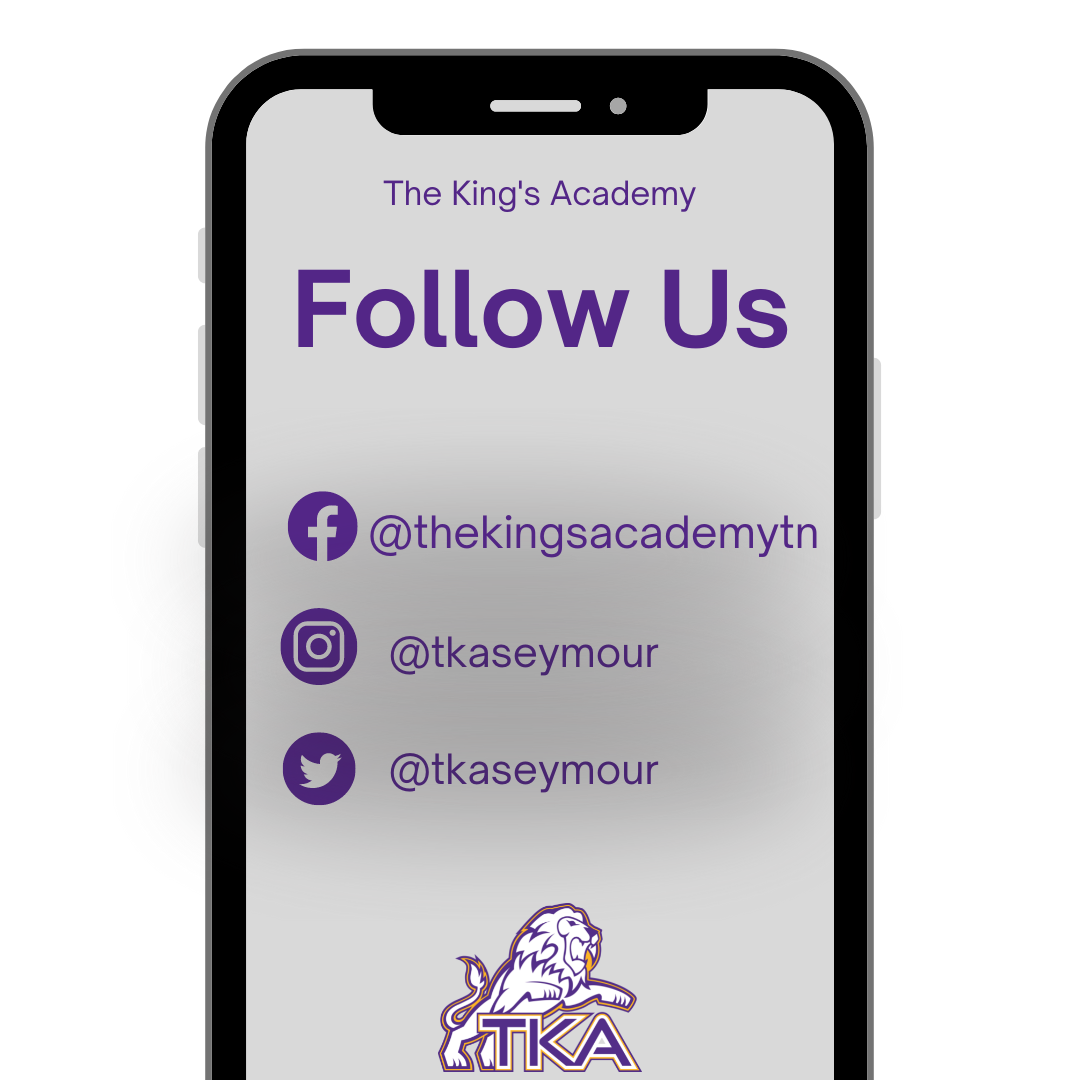table div table+table+table+table+table+table+table+table+table+table+table+table+table+table+table+table+table+table+table+table+table+table+table+table+table+table+table+table+table+table+table+table+table+table+table+table+table+table+table+table+table+table+table+table+table+table+table+table+table+table+table+table+table+table+table+table+table+table+table+table+table+table+table+table+table div table{width:100%;padding:0}table div table+table+table+table+table+table+table+table+table+table+table+table+table+table+table+table+table+table+table+table+table+table+table+table+table+table+table+table+table+table+table+table+table+table+table+table+table+table+table+table+table+table+table+table+table+table+table+table+table+table+table+table+table+table+table+table+table+table+table+table+table+table+table+table+table div table img{width:96.23%;padding:0;float:none}table div table+table+table+table+table+table+table+table+table+table+table+table+table+table+table+table+table+table+table+table+table+table+table+table+table+table+table+table+table+table+table+table+table+table+table+table+table+table+table+table+table+table+table+table+table+table+table+table+table+table+table+table+table+table+table+table+table+table+table+table+table+table+table+table+table div table td{width:100%;padding:0 1.88% 18px}/* styles *//* styles */ The King's Academy
 Like   Tweet